# Vibration of Simply Supported Laminated PlatesThe purpose of this test is to check laminated plates with orthotropic materials.
You will use 2D meshes.Reference:

Mechanics of Composite Materials, Robert M. Jones, Hemisphere Publishing Corporation, chap 5.5.1, p270.

##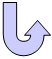Specifications

### Geometry Specifications

 a = 0.1 m b = 0.1 m t1 = t3 = 0.0001 m t2 = 0.0004 m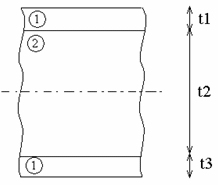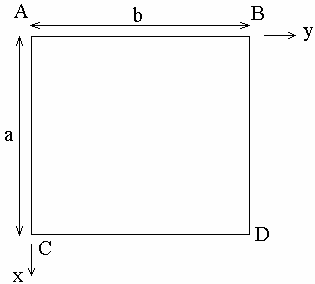### Analysis Specifications

 Material 1: Young Modulus (material):E1 = 2 x 1011 Pa E2 = 2 x 1010 Pa Poisson's Ratio: ν12 = 0.3  Shearing coefficient G12 = G23 = G13 = 5.169 x 109 Pa Density: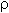= 6000 kg x m-3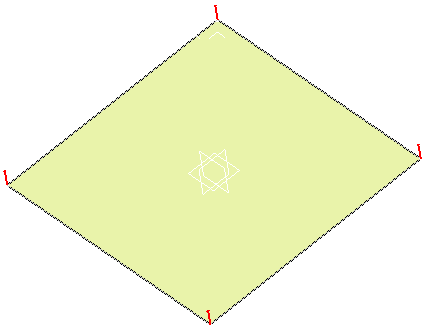Material 2: Young Modulus (material):E1 = 7 x 1010 Pa E2 = 7 x 109 Pa Poisson's Ratio: ν12 = 0.2  Shearing coefficient G12 = G23 = G13 = 3.203 x 109 Pa Density:= 6000 kg x m-3 Mesh Specifications: 2D mesh with linear quadrangle elements (QD4) Mesh size: 2 mm Restraints (User-defined): On AB, CD, AC and BD: translation along Z = 0

##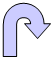Results

### Analytical Results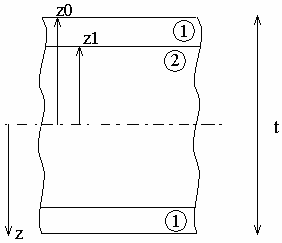For a simply supported plate, we have: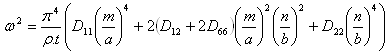where: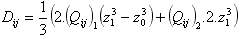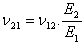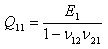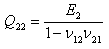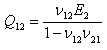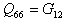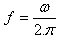Conditions for a specially orthotropic material are:

• D11 / D22 = 10

• D12 + 2D66 = 1

m: transversal vibration mode
n: longitudinal vibration mode

### Computed Results

 Modes Reference results [Hz] Computed results [Hz] Normalized results Visualization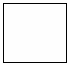m = 1 n = 1 242.724 242.16 0.997676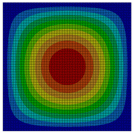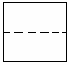m = 1 n = 2 390.785 389.992 0.997971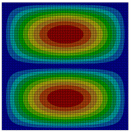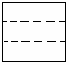m = 1 n = 3 702.403 701.991 0.999413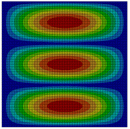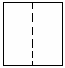m = 2 n = 1 880.240 874.553 0.99354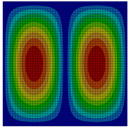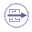To Perform the Test:

The orthotropic_laminates.CATAnalysis document presents a complete analysis of this case.

To compute the case, proceed as follow:

1. Open the CATAnalysis document.

2. Compute the case in the Generative Structural Analysis workbench.